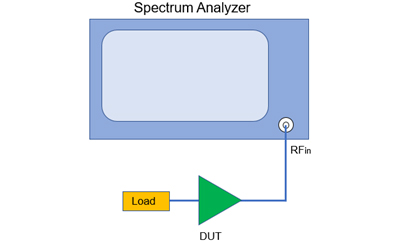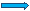# 噪声系数测试之增益法

2020-01-03 来源：微波射频网作者：Knight 我要评论(0) 字号：N0(dBm) = -174dBm/Hz + 10lg(B) + G(dB) + NF

N0(dBm) = -174dBm/Hz + 10lg(RBW) + G(dB) + NF

 N0(dBm) - 10lg(RBW) = -174dBm/Hz + G(dB) + NF

“=”左边的式子即为频谱仪上测得的噪声功率谱密度，这个参数频谱仪可以直接测出。DUT的增益已知，即可计算出其噪声系数NF。

N0 = kBTin*G + kBT*G

Tin = α*T0，    T = β*T0

N0 = kB*α*T0*G + kB*β*T0*G = kBT0*G*(α + β)

N0(dBm) = -174dBm/Hz + 10lg(RBW) + G(dB) + 10lg(α + β)

 N0(dBm) - 10lg(RBW) = -174dBm/Hz + G(dB) + 10lg(α + β)

NF = 10lg(1+T/T0)

Ntotal = kBT0*G*F + kBTSA*GSA

Ntotal = kBT0*G*F + kBTSA

 FSA – 1 << G*F(FSA – 1) / GF << 1

Ftotal = F + (FSA - 1) / G

Ftotal / F = 1 + (FSA - 1) / GF

NFtotal – NF = 10lg[1 + (FSA-1) / GF]

NF = NFtotal - ∆NF

 (FSA – 1) / GF 0.1 0.05 0.01 Ftotal / F 1.1 1.05 1.01 ∆NF(dB) 0.414 0.212 0.043

 (FSA -1) / GF ≤0.05

(FSA -1) / GF = (10-1) / (1000*1.413) ≈0.006

(1)    增益法需要频谱仪而不需要噪声头，因此无法测试DUT的增益，需要DUT增益已知。
(2)    增益法忽略了频谱仪自身的噪声系数，测试结果是DUT与频谱仪的级联噪声系数。为了提高测试精度，建议测试增益相对较高的DUT时再使用增益法。
(3)    如果频谱仪配置了预放大器，使用增益法测试时，要根据DUT的增益大小来决定是否需要打开预放大器。并不是打开预放大器一定可以提高测试精度，也要防止频谱仪饱和！
(4)    测试时，一定要根据当前室温是否是T0来确定选择相应的公式进行计算，否则将会带来测试精度的恶化。
(5)    如果可以确定频谱仪的噪声系数，则也可以根据上述公式从增益法测得的结果中消除频谱仪带来的影响，从而得到待测件本身的噪声系数。
 微波射频行业人士 | 相聚在这里【10大细分领域的微信技术交流群】订阅微信公众号加群管理员为好友